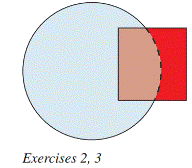Chapter 8.1, Problem 2E### Elementary Geometry for College St...

6th Edition
Daniel C. Alexander + 1 other
ISBN: 9781285195698

#### Solutions

Chapter
Section### Elementary Geometry for College St...

6th Edition
Daniel C. Alexander + 1 other
ISBN: 9781285195698
Textbook Problem
1 views

# The area of the square is 12, and the area of the circle is 30. Does the area of the entire shaded region equal 42? Why or why not?To determine

Whether the area of the entire shaded region is equal to 42?

Explanation

Given,

The area of the square =12 unit2

The area of the circle =30 unit2

From the figure,

The area of the circle =12

x+z=12(1)

z=12x

The area of the square =30

x+y=30(2)

y=30x

The area of the entire shaded region =42

x+y+z=42(3)

If x,y,z0

Substitute y and z value in equation (s)

x+y+<

### Still sussing out bartleby?

Check out a sample textbook solution.

See a sample solution

#### The Solution to Your Study Problems

Bartleby provides explanations to thousands of textbook problems written by our experts, many with advanced degrees!

Get Started

#### In Exercises 49-62, find the indicated limit, if it exists. 54. limb3b+1b+3

Applied Calculus for the Managerial, Life, and Social Sciences: A Brief Approach

#### True or False: A straight (simple) substitution may be used to evaluate 2+lnxxdx.

Study Guide for Stewart's Single Variable Calculus: Early Transcendentals, 8th

#### True or False: converges.

Study Guide for Stewart's Multivariable Calculus, 8th

#### Graph each function. fx=2x+52-8

College Algebra (MindTap Course List)